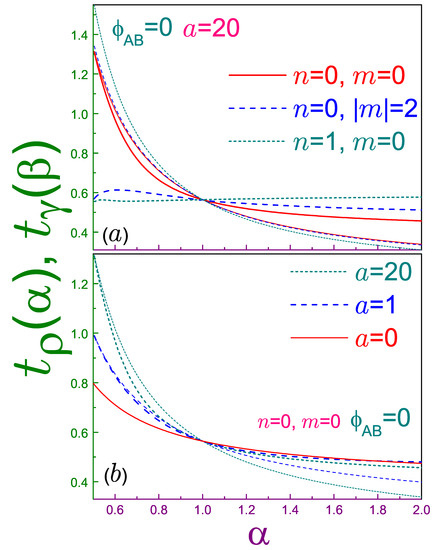Next Article in Journal
Related Entropy Theories Application in Condition Monitoring of Rotating Machineries
Previous Article in Journal
Lagrangian for Circuits with Higher-Order Elements
Previous Article in Special Issue
On Data-Processing and Majorization Inequalities for f-Divergences with Applications
Open AccessArticle

# Rényi and Tsallis Entropies of the Aharonov–Bohm Ring in Uniform Magnetic Fields

byOleg OlendskiDepartment of Applied Physics and Astronomy, University of Sharjah, P.O. Box, Sharjah 27272, UAE
Entropy 2019, 21(11), 1060; https://doi.org/10.3390/e21111060
Received: 7 October 2019 / Revised: 24 October 2019 / Accepted: 26 October 2019 / Published: 29 October 2019
One-parameter functionals of the Rényi $R ρ , γ ( α )$ and Tsallis $T ρ , γ ( α )$ types are calculated both in the position (subscript $ρ$ ) and momentum ( $γ$ ) spaces for the azimuthally symmetric 2D nanoring that is placed into the combination of the transverse uniform magnetic field $B$ and the Aharonov–Bohm (AB) flux $ϕ A B$ and whose potential profile is modeled by the superposition of the quadratic and inverse quadratic dependencies on the radius r. Position (momentum) Rényi entropy depends on the field B as a negative (positive) logarithm of $ω e f f ≡ ω 0 2 + ω c 2 / 4 1 / 2$ , where $ω 0$ determines the quadratic steepness of the confining potential and $ω c$ is a cyclotron frequency. This makes the sum $R ρ n m ( α ) + R γ n m ( α 2 α − 1 )$ a field-independent quantity that increases with the principal n and azimuthal m quantum numbers and satisfies the corresponding uncertainty relation. In the limit $α → 1$ , both entropies in either space tend to their Shannon counterparts along, however, different paths. Analytic expression for the lower boundary of the semi-infinite range of the dimensionless coefficient $α$ where the momentum entropies exist reveals that it depends on the ring geometry, AB intensity, and quantum number m. It is proved that there is the only orbital for which both Rényi and Tsallis uncertainty relations turn into the identity at $α = 1 / 2$ , which is not necessarily the lowest-energy level. At any coefficient $α$ , the dependence of the position of the Rényi entropy on the AB flux mimics the energy variation with $ϕ A B$ , which, under appropriate scaling, can be used for the unique determination of the associated persistent current. Similarities and differences between the two entropies and their uncertainty relations are discussed as well. View Full-Text
Show FiguresFigure 1

MDPI and ACS Style

Olendski, O. Rényi and Tsallis Entropies of the Aharonov–Bohm Ring in Uniform Magnetic Fields. Entropy 2019, 21, 1060.